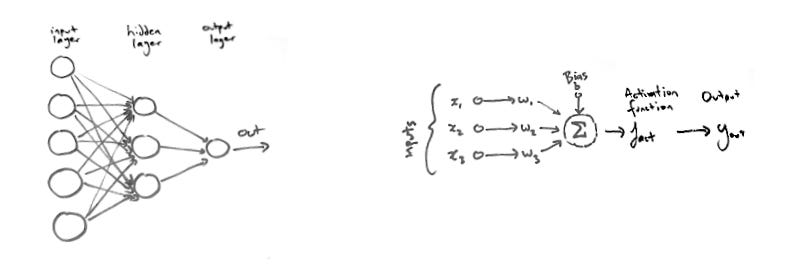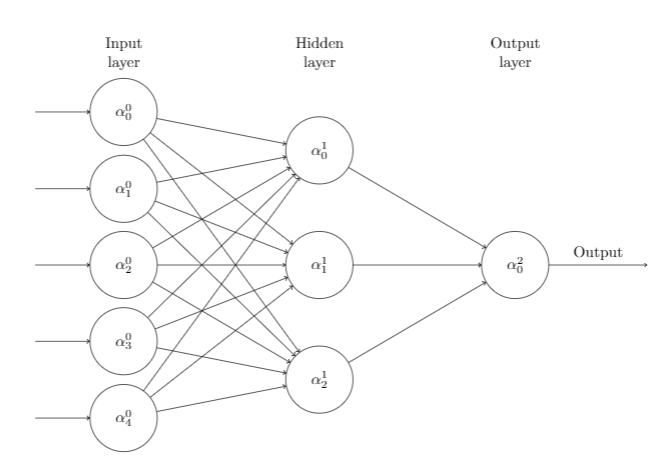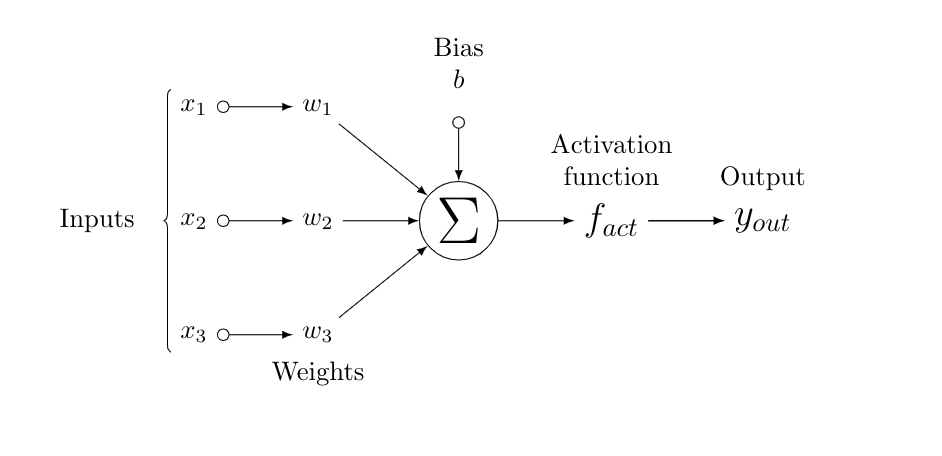# Typesetting neural network diagrams with TeXPhoto by Raphael Schaller on Unsplash

TeX is the lingua franca of scientific publishing. It is powerful and precise, a 70’s throwback that is a testament to its creator’s Donald Knuth genius. Whilst TeX is great, it can be very complex once you start doing more than just simple typesetting and there is not a lot of documentation to help.

I wanted to use TeX to typeset some diagrams of neural networks for an upcoming paper. Nothing too complex, a few symbols and a number of circles with directed lines connecting them, see Figure 1 below. I managed to ‘TeX’ the diagrams and was happy with the results, however it was a non-trivial process. This story is to share the solution and help out anyone looking to typeset neural networks with TeX.Figure 1: Diagrams of neural network layers and pipeline

I used the TikZ package to typeset the diagrams. Tikz is a powerful but complex graphics package for TeX. It is a set of high level macros built with PGF, a low level language for producing vector graphics from a geometric/algebraic descriptions. The manual for TikZ/PGF is around 1280 pages and this gallery has a number of examples of what is possible with TikZ.

To get started with TikZ, you need to instruct TeX to use the Tikz package and the load Tikz libraries in the document preamble:

\usepackage{tikz}\usetikzlibrary{matrix,chains,positioning,decorations.pathreplacing,arrows}

Once the preamble is configured, you can declare tikzpicture environments with the description of the diagram to be drawn. The tikzpicture environment can be enclosed inside a figure or similar environment.

TikZ offers two libraries that make positioning easier when arranging a large number of nodes: the matrix library and the chains library. The first allows you to arrange multiple nodes in rows and columns, while the second is mostly useful for creating “chains of nodes” and, more generally “flows”.

Listing 1 below, contains the code to generate the first diagram. We use the matrix library to build a 10 by 3 matrix to hold the nodes. There are 3 columns, one for each of the layers and 10 rows; one for the headings, 5 for neurons in the first column and four blank for spacing. We use ‘|[clear]|’ to remove the default net styling on the matrix cells. After the matrix is defined we draw lines between the neurons, by using for example:

draw[->] (mat-\ai-1) — (mat-\aii-2);

This draws a directed arrow -> between the matrix’s cells in row \ai column 1 and row \aii column 2. Here \ai and \aii are loop variables with index references for the matrix being 1 based.

% Listing 1: Tex for neural network layers
\begin{tikzpicture}[     % define styles      clear/.style={          draw=none,         fill=none     },     net/.style={         matrix of nodes,         nodes={ draw, circle, inner sep=10pt },         nodes in empty cells,         column sep=2cm,         row sep=-9pt     },     >=latex]
% define matrix mat to hold nodes
% using net as default style for cells\matrix[net] (mat){% Define layer headings|[clear]| \parbox{1.3cm}{\centering Input\\layer}     & |[clear]| \parbox{1.3cm}{\centering Hidden\\layer}     & |[clear]| \parbox{1.3cm}{\centering Output\\layer} \\         $\alpha_{0}^{0}$  & |[clear]|        & |[clear]| \\|[clear]|         & $\alpha_{0}^{1}$ & |[clear]| \\$\alpha_{1}^{0}$  & |[clear]|        & |[clear]| \\|[clear]|         & |[clear]|        & |[clear]| \phantom{$a_{0}^{0}$} \\$\alpha_{2}^{0}$  & $\alpha_{1}^{1}$ & $\alpha_{0}^{2}$ \\|[clear]|         & |[clear]|        & |[clear]|  \phantom{$a_{0}^{0}$} \\$\alpha_{3}^{0}$  & |[clear]|        & |[clear]| \\|[clear]|         & $\alpha_{2}^{1}$ & |[clear]| \\$\alpha_{4}^{0}$  & |[clear]|        & |[clear]| \\ };
% left most lines into input layers\foreach \ai in {2,4,...,10}    \draw[<-] (mat-\ai-1) -- +(-2cm,0);
% lines from a_{i}^{0} to each a_{j}^{1}\foreach \ai in {2,4,...,10} {    \foreach \aii in {3,6,9}        \draw[->] (mat-\ai-1) -- (mat-\aii-2);        }
% lines from a_{i}^{1} to a_{0}^{2}\foreach \ai in {3,6,9}  \draw[->] (mat-\ai-2) -- (mat-6-3);    % right most line with Output label\draw[->] (mat-6-3) -- node[above] {Output} +(2cm,0);
\end{tikzpicture}

The output for the above code is shown in Figure 2:Figure 2: Output for neural network layers diagram

Listing 2 contains the code to generate the second diagram. As for the first diagram, we start by defining the styles. However rather than using the matrix library, we will use Tikz chain’s library and create 3 chains for each path or flow through the pipeline.

% Listing 2: Tex for neural network pipeline
\begin{tikzpicture}[    % define styles        init/.style={          draw,          circle,          inner sep=2pt,         font=\Huge,         join = by -latex    },    squa/.style={         font=\Large,        join = by -latex    }]
% Top chain x1 to w1
\begin{scope}[start chain=1]    \node[on chain=1] at (0,1.5cm)  (x1) {$x_1$};    \node[on chain=1,join=by o-latex] (w1) {$w_1$};\end{scope}
% Middle chain x2 to output
\begin{scope}[start chain=2]    \node[on chain=2] (x2) {$x_2$};    \node[on chain=2,join=by o-latex] {$w_2$};    \node[on chain=2,init] (sigma) {$\displaystyle\Sigma$};    \node[on chain=2,squa,label=above:{\parbox{2cm}{\centering Activation\\ function}}]   {$f_{act}$};    \node[on chain=2,squa,label=above:Output,join=by -latex] {$y_{out}$};\end{scope}
% Bottom chain x3 to w3
\begin{scope}[start chain=3]    \node[on chain=3] at (0,-1.5cm)     (x3) {$x_3$};    \node[on chain=3,label=below:Weights,join=by o-latex]    (w3) {$w_3$};\end{scope}
% Bias
\node[label=above:\parbox{2cm}{\centering Bias \\ $b$}] at (sigma|-w1) (b) {};
% Arrows joining w1, w3 and b to sigma
\draw[-latex] (w1) -- (sigma);\draw[-latex] (w3) -- (sigma);\draw[o-latex] (b) -- (sigma);
% left hand side brace\draw[decorate,decoration={brace,mirror}] (x1.north west) -- node[left=10pt] {Inputs} (x3.south west);
\end{tikzpicture}

The output for the above code is shown in Figure 3:Figure 3: Output for neural network pipeline diagram

The complete code is available on GitHub: https://github.com/dreading/tex-neural-network.